• No products in the cart.

# 104.3.5 Box Plots and Outlier Detection using Python

##### Basics of a box plot.
In this post, we will discuss a basics or boxplots and how they help us identify outliers.
We will be carrying same python session form series 104 blog posts, i.e. same datasets.

### Box plots and Outlier Detection

• Box plots have box from LQ to UQ, with median marked.
• They portray a five-number graphical summary of the data Minimum, LQ, Median, UQ, Maximum
• Helps us to get an idea on the data distribution
• Helps us to identify the outliers easily
• 25% of the population is below first quartile,
• 75% of the population is below third quartile
• If the box is pushed to one side and some values are far away from the box then it’s a clear indication of outliers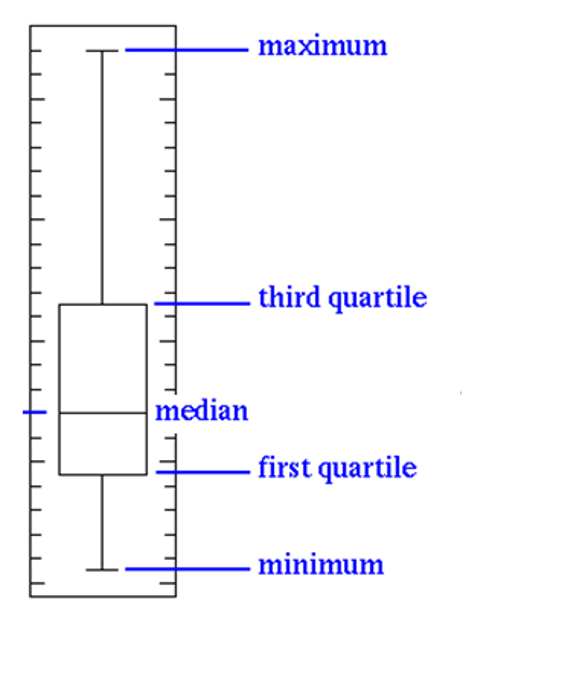• Some set of values far away from box,  gives us a clear indication of outliers.
• In this example the minimum is 5, maximum is 120, and 75% of the values are less than 15.
• Still there are some records reaching 120. Hence a clear indication of outliers.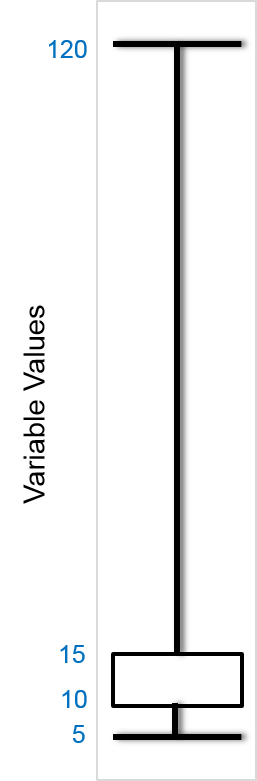• Sometimes the outliers are so evident that, the box appear to be a horizontal line in box plot.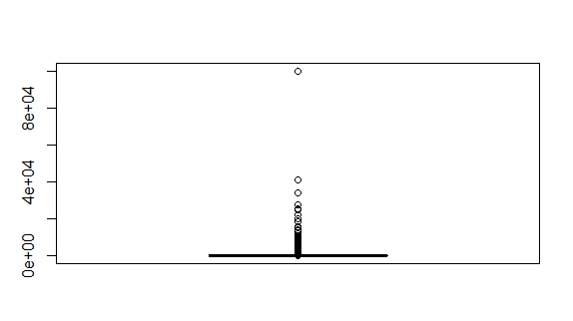### Box plots and outlier detection on Python

In :
```import numpy as np
import matplotlib.pyplot as plt

%matplotlib inline

plt.boxplot(bank.balance)
```
Out:
```{'boxes': [<matplotlib.lines.Line2D at 0xcbcd400>],
'caps': [<matplotlib.lines.Line2D at 0xcbdde10>,
<matplotlib.lines.Line2D at 0xcbddf28>],
'fliers': [<matplotlib.lines.Line2D at 0xccc4f98>],
'means': [],
'medians': [<matplotlib.lines.Line2D at 0xccc4780>],
'whiskers': [<matplotlib.lines.Line2D at 0xcbcdda0>,
<matplotlib.lines.Line2D at 0xcbcdeb8>]}```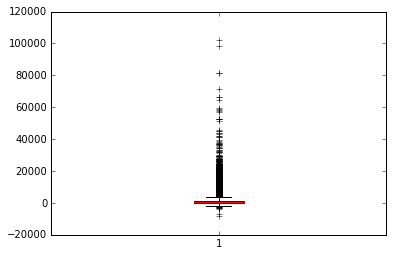### Practice: Box plots and outlier detection

• Dataset: “./Bank Marketing/bank_market.csv”
• Draw a box plot for balance variable
• Do you suspect any outliers in balance ?
• Get relevant percentiles and see their distribution.
• Draw a box plot for age variable
• Do you suspect any outliers in age?
• Get relevant percentiles and see their distribution.
In :
```plt.boxplot(bank.balance)
```
Out:
```{'boxes': [<matplotlib.lines.Line2D at 0xcc78208>],
'caps': [<matplotlib.lines.Line2D at 0xcc7fc18>,
<matplotlib.lines.Line2D at 0xcc7fd30>],
'fliers': [<matplotlib.lines.Line2D at 0xcc84da0>],
'means': [],
'medians': [<matplotlib.lines.Line2D at 0xcc84588>],
'whiskers': [<matplotlib.lines.Line2D at 0xcc78ba8>,
<matplotlib.lines.Line2D at 0xcc78cc0>]}```outlier are present in balance variable

In :
```#Get relevant percentiles and see their distribution
bank['balance'].quantile([0, 0.1, 0.2, 0.3, 0.4, 0.5, 0.6, 0.7, 0.8, 0.9, 1])
```
Out:
```0.0     -8019.0
0.1         0.0
0.2        22.0
0.3       131.0
0.4       272.0
0.5       448.0
0.6       701.0
0.7      1126.0
0.8      1859.0
0.9      3574.0
1.0    102127.0
Name: balance, dtype: float64```
In :
```# Draw a box plot for age variable
plt.boxplot(bank.age)
```
Out:
```{'boxes': [<matplotlib.lines.Line2D at 0xcf54470>],
'caps': [<matplotlib.lines.Line2D at 0xcf5be80>,
<matplotlib.lines.Line2D at 0xcf5bf98>],
'fliers': [<matplotlib.lines.Line2D at 0xcf65748>],
'means': [],
'medians': [<matplotlib.lines.Line2D at 0xcf617f0>],
'whiskers': [<matplotlib.lines.Line2D at 0xcf54e10>,
<matplotlib.lines.Line2D at 0xcf54f28>]}```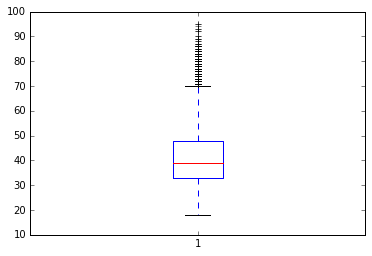No outliers are present

In :
```#Get relevant percentiles and see their distribution
bank['age'].quantile([0, 0.1, 0.2, 0.3, 0.4, 0.5, 0.6, 0.7, 0.8, 0.9, 1])
```
Out:
```0.0    18.0
0.1    29.0
0.2    32.0
0.3    34.0
0.4    36.0
0.5    39.0
0.6    42.0
0.7    46.0
0.8    51.0
0.9    56.0
1.0    95.0
Name: age, dtype: float64

```

Next post is about creating graphs in python.
Link to the next post :https://statinfer.com/104-3-6-creating-graphs-in-python/

24th January 2018